# 利用箱形图（Boxplot）探索金融时间序列的季节性形态

### 尝试驳斥有效市场假说，并证明市场周期的存在

2013 年，尤金·法玛（Eugene Fama）开发了有效市场假设，并获得了诺贝尔经济学奖。 根据他的假设，资产价格能够完全反映所有重要信息。 这意味着没有任何一个市场参与者会比其人更具有优势。

• 如果市场资产价格充分反映了有关该资产的过去信息，则偏弱
• 当价格不仅反映过去，而且反映了当前公开信息，则较为平均
• 当它还反映了非公开的内部信息时，则偏强

### 在价格增幅中搜索季节性形态

```from MetaTrader5 import *
from datetime import datetime
import numpy as np
import pandas as pd
import matplotlib.pyplot as plt
%matplotlib inline
import seaborn; seaborn.set()
# Initializing MT5 connection
MT5WaitForTerminal()

print(MT5TerminalInfo())
print(MT5Version())

```

```rates = pd.DataFrame(MT5CopyRatesRange("EURUSD", MT5_TIMEFRAME_D1, datetime(2010, 1, 1), datetime(2020, 1, 1)),
columns=['time', 'open', 'low', 'high', 'close', 'tick_volume', 'spread', 'real_volume'])
# leave only 'time' and 'close' columns
rates.drop(['open', 'low', 'high', 'tick_volume', 'spread', 'real_volume'], axis=1)

# get percent change (price returns)
returns = pd.DataFrame(rates['close'].pct_change(1))
returns = returns.set_index(rates['time'])
returns = returns[1:]

Monthly_Returns = returns.groupby([returns.index.year.rename('year'), returns.index.month.rename('month')]).mean()
Monthly_Returns.boxplot(column='close', by='month', figsize=(15, 8))

```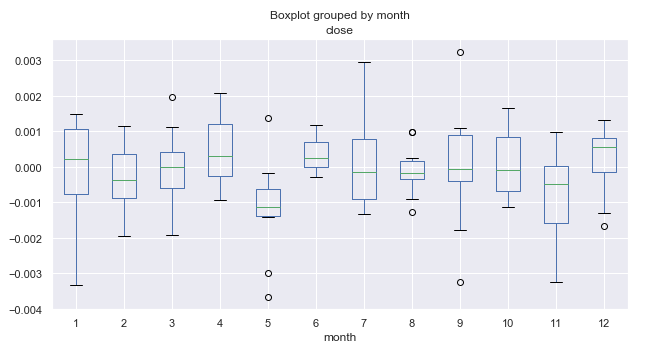### 什么是箱形图，以及如何解释它们？

1. 中位数，Q2 或第 50 的百分数显示数据集的平均值。 该值在图例中的箱内以绿色水平线示意。
2. 第一个四分位数，Q1（或第 25 的百分数）代表 Q2 和样本中最小值之间的中位数，该中位数落在 99％ 置信区间内。 它在图中显示为箱子的“实体”下边缘。
3. 第三个四分位数，Q3（或第 75 的百分数）是 Q2 和最大值之间的中位数，显示为箱子的“实体”上边缘。
4. 箱子的实体形成了四分位间距（介于 25％ 和 75％ 之间），也称为 IQR。
5. 箱子的须则充实了分布。 它们覆盖了整个样本的 99％，上方和下方的点表示超出 99％ 范围的数值。

### 进一步分析季节性形态

`Daily_Returns = returns.groupby([returns.index.week.rename('week'), returns.index.dayofweek.rename('day')]).mean()`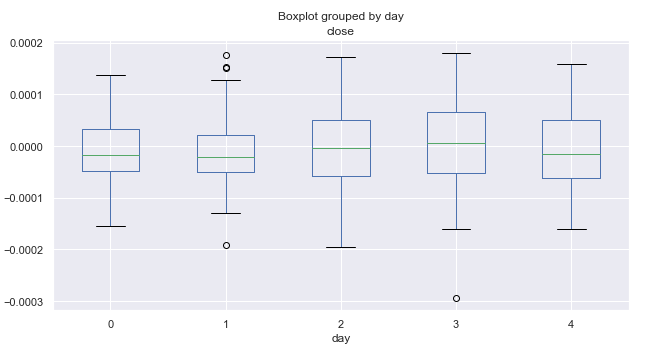```# leave only one month "returns.index[~returns.index.month.isin()"
returns = returns.drop(returns.index[~returns.index.month.isin()])

```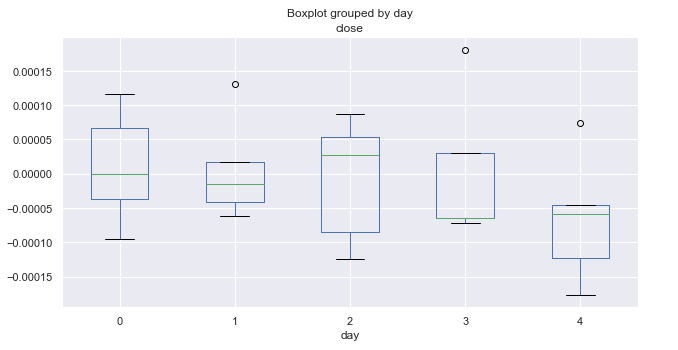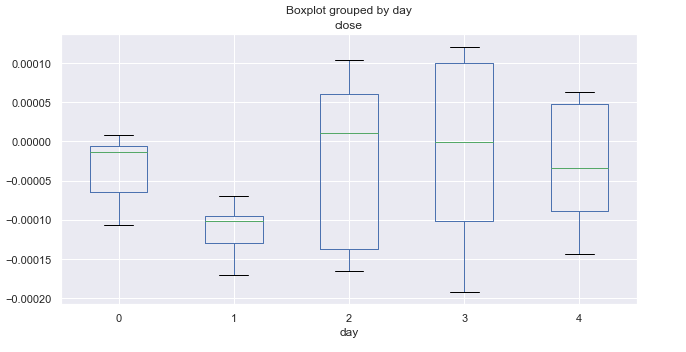图例 4. 按交易日的平均价格增幅，覆盖 10 年（三月份）。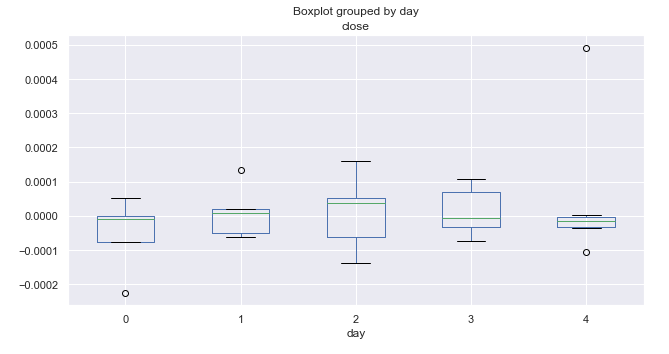### 季节性分析日内形态

```rates = pd.DataFrame(MT5CopyRatesRange("EURUSD", MT5_TIMEFRAME_M15, datetime(2010, 1, 1), datetime(2019, 11, 25)),
columns=['time', 'open', 'low', 'high', 'close', 'tick_volume', 'spread', 'real_volume'])
# leave only 'time' and 'close' columns
rates.drop(['open', 'low', 'high', 'tick_volume', 'spread', 'real_volume'], axis=1)

# get percent change (price returns)
returns = pd.DataFrame(rates['close'].pct_change(1))
returns = returns.set_index(rates['time'])
returns = returns[1:]

Hourly_Returns = returns.groupby([returns.index.day.rename('day'), returns.index.hour.rename('hour')]).median()
Hourly_Returns.boxplot(column='close', by='hour', figsize=(10, 5))

```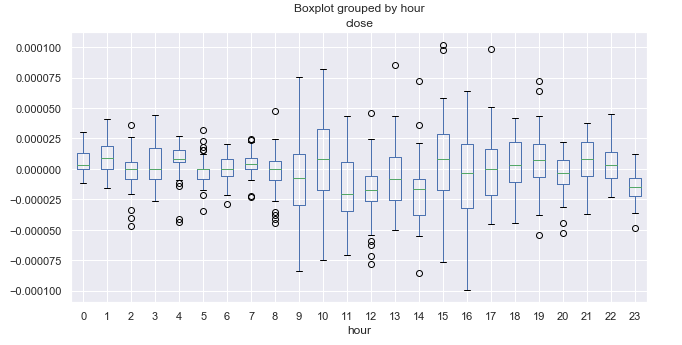### 按均线（MA）搜索去趋势化季节性形态

```rates = pd.DataFrame(MT5CopyRatesRange("EURUSD", MT5_TIMEFRAME_M15, datetime(2010, 1, 1), datetime(2019, 11, 25)),
columns=['time', 'open', 'low', 'high', 'close', 'tick_volume', 'spread', 'real_volume'])
# leave only 'time' and 'close' columns
rates = rates.drop(['open', 'low', 'high', 'tick_volume', 'spread', 'real_volume'], axis=1)
rates = rates.set_index('time')
# set the moving average period
window = 25
# detrend tome series by MA
ratesM = rates.rolling(window).mean()
ratesD = rates[window:] - ratesM[window:]

plt.figure(figsize=(10, 5))
plt.plot(rates)
plt.plot(ratesM)

```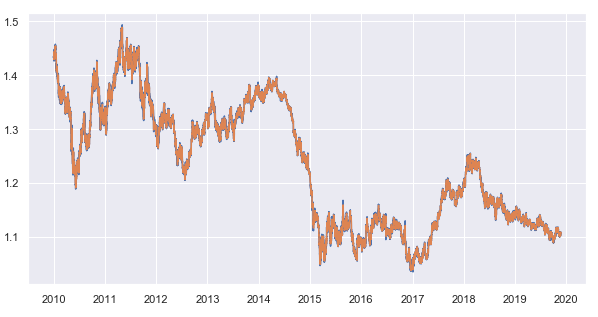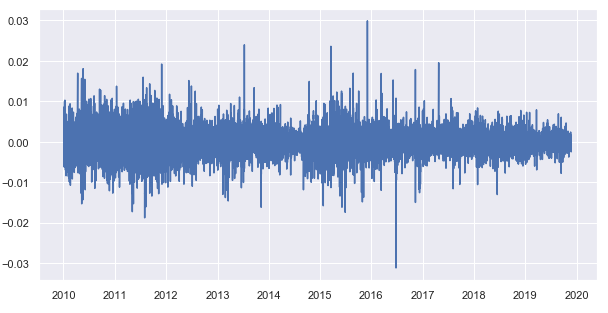```Hourly_Returns = ratesD.groupby([ratesD.index.day.rename('day'), ratesD.index.hour.rename('hour')]).median()
Hourly_Returns.boxplot(column='close', by='hour', figsize=(15, 8))

```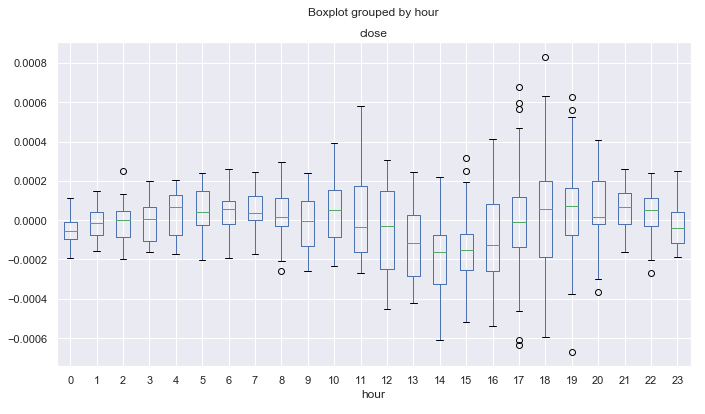`Hourly_std = ratesD.groupby([ratesD.index.day.rename('day'), ratesD.index.hour.rename('hour')]).std()`

###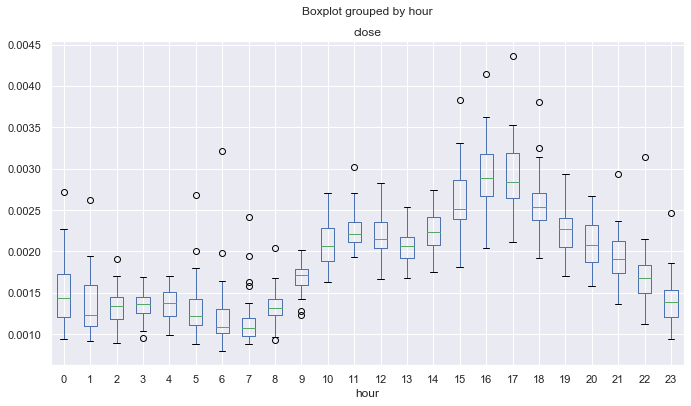```Hourly_skew = ratesD.groupby([ratesD.index.day.rename('day'), ratesD.index.hour.rename('hour')]).skew()

```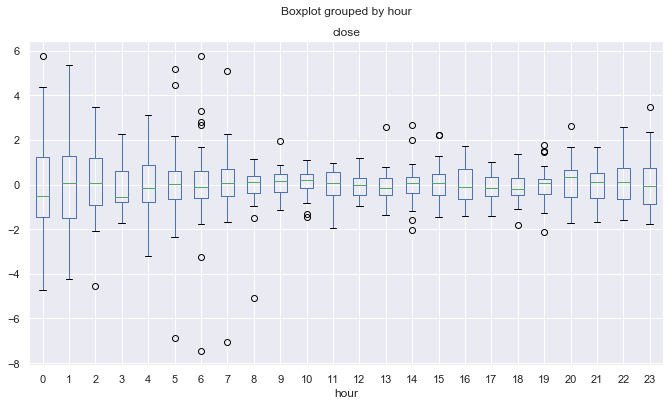```Hourly_std = ratesD.groupby([ratesD.index.day.rename('day'), ratesD.index.hour.rename('hour')]).apply(pd.DataFrame.kurt)

```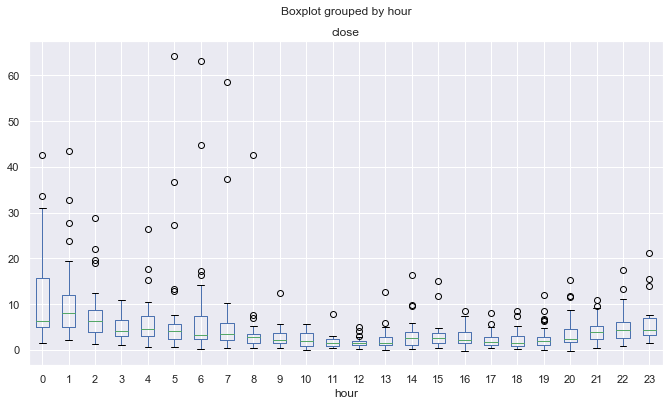### 搜索特定月份或一周中某天的季节性形态，按均线去趋势化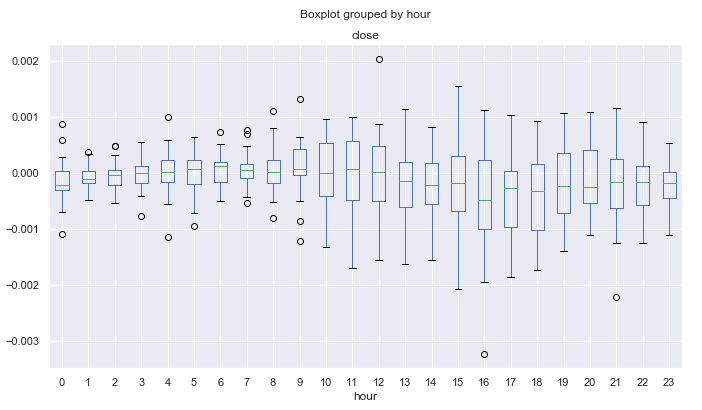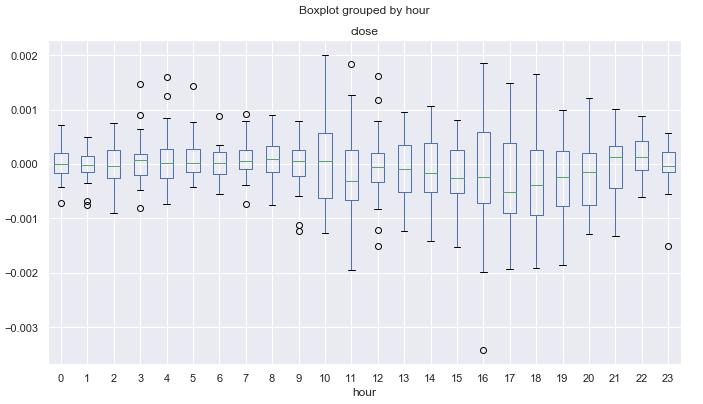### 利用交易逻辑检查形态

```//+------------------------------------------------------------------+
//|                                  Copyright 2020, Max Dmitrievsky |
//|                        https://www.mql5.com/en/users/dmitrievsky |
//+------------------------------------------------------------------+
#property version   "1.00"

#include <MT4Orders.mqh>
#include <Math\Stat\Math.mqh>

input int OrderMagic = 666;
input double   MaximumRisk=0.01;
input double   CustomLot=0;

int hnd = iMA(NULL, 0, 25, 0, MODE_SMA, PRICE_CLOSE);
MqlDateTime hours;
double maArr[], prArr[];

void OnTick()
{
//---
CopyBuffer(hnd, 0, 0, 1, maArr);
CopyClose(NULL, 0, 0, 1, prArr);
double pr = prArr - maArr;

TimeToStruct(TimeCurrent(), hours);
if(hours.hour >=0 && hours.hour <=4)
if(countOrders(0)==0 && countOrders(1)==0)

if(countOrders(0)!=0 && pr >=0)
for(int b=OrdersTotal()-1; b>=0; b--)
if(OrderSelect(b,SELECT_BY_POS)==true && OrderMagicNumber() == OrderMagic) {
if(OrderClose(OrderTicket(),OrderLots(),OrderClosePrice(),0,Red)) {};
}

}
//+------------------------------------------------------------------+
//|                                                                  |
//+------------------------------------------------------------------+
int countOrders(int a) {
int result=0;
for(int k=0; k<OrdersTotal(); k++) {
if(OrderType()==a && OrderMagicNumber()==OrderMagic && OrderSymbol() == _Symbol) result++;
}
return(result);
}

```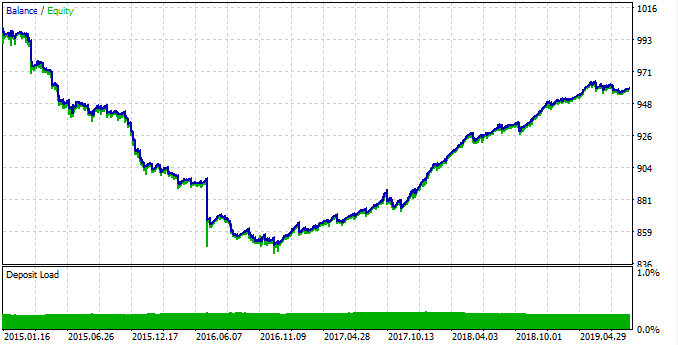```rates = pd.DataFrame(MT5CopyRatesRange("EURUSD", MT5_TIMEFRAME_M15, datetime(2017, 1, 1), datetime(2019, 11, 25)),
columns=['time', 'open', 'low', 'high', 'close', 'tick_volume', 'spread', 'real_volume'])

```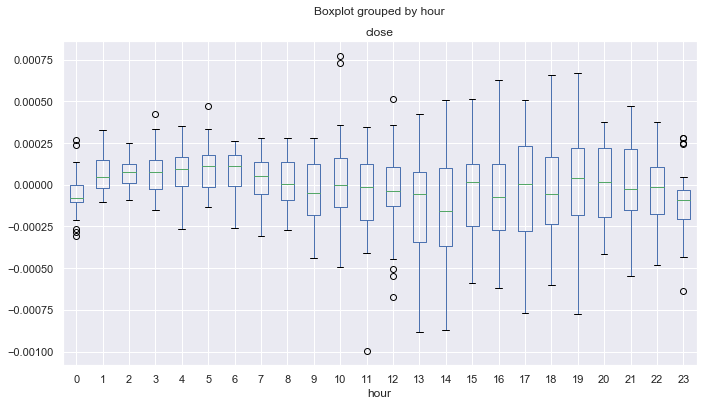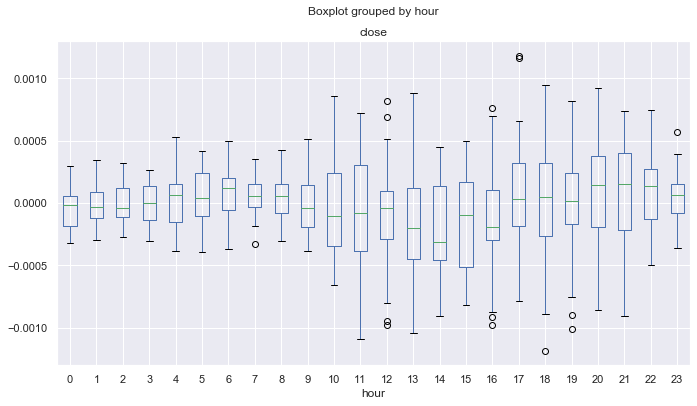```TimeToStruct(TimeCurrent(), hours);
if(hours.hour >=0 && hours.hour <=1)
if(countOrders(0)==0 && countOrders(1)==0)

if(countOrders(0)!=0 && pr >= 0.0003)
for(int b=OrdersTotal()-1; b>=0; b--)
if(OrderSelect(b,SELECT_BY_POS)==true && OrderMagicNumber() == OrderMagic) {
if(OrderClose(OrderTicket(),OrderLots(),OrderClosePrice(),0,Red)) {};
}

```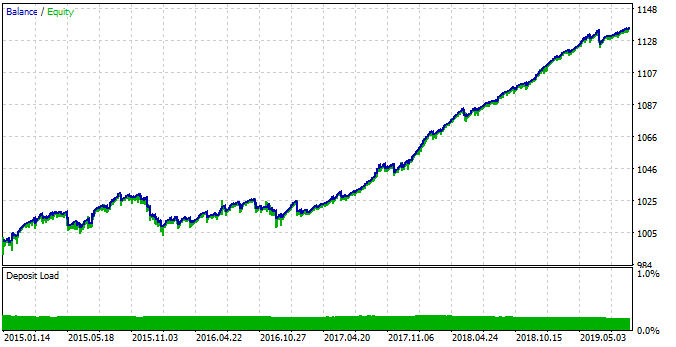## 量化课程

移动端课程